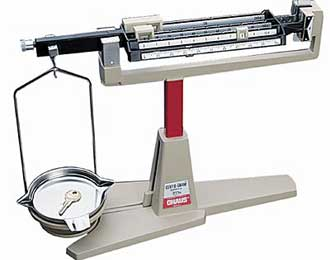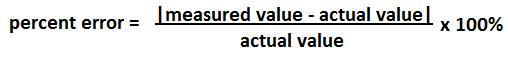﻿ Percent Error Calculator ﻿# Percent Error CalculatorMeasured Value Actual Value Percent Error:

This percent error calculator calculates the error percentage that a measured value is off from its actucal value.

The measured value represents the value which a person obtains from measuring using any type of instrument or resource available. Being that a person manually obtains this value, it is subject to error. For example, when weighing a device using a centigram balance, the person may find the weight without the balance being perfectly centered. Thus, the person will record the weight of the item with a certain percent error.

On the other hand, the actual, or real, value is the true value of the item. This would represent the actual value of the item, not the value recorded experimentally.

When we take the measured value and subtract the actual value from it, and then divide by the actual value, multiplied by 100%, we obtain the error percentage. This error percent measures how many percent the measured value is off from the actual or real value.

The smaller the error percentage, the more accurate or close the measured value is to the actual value. The greater the percent error, the farther the measured value is from its real value.

To use this calculator, a user just has to enter the measured value and the actual value and then click the 'Calculate' button. The resultant percent error value will then be automatically computed and shown.

The percent error is used in all different types of scientific settings, including electronics.

Example Calculations

What is the percent error when the measured value is 85 and the actual value is 100?